# The MI Procedure

### Producing Monotone Missingness with the MCMC Method

Subsections:

The monotone data MCMC method was first proposed by Li (1988) and Liu (1993) described the algorithm. The method is useful especially when a data set is close to having a monotone missing pattern. In this case, the method needs to impute only a few missing values to the data set to have a monotone missing pattern in the imputed data set. Compared to a full data imputation that imputes all missing values, the monotone data MCMC method imputes fewer missing values in each iteration and achieves approximate stationarity in fewer iterations (Schafer, 1997, p. 227).

You can request the monotone MCMC method by specifying the option IMPUTE=MONOTONE in the MCMC statement. The "Missing Data Patterns" table now denotes the variables with missing values by "." or "O". The value "." means that the variable is missing and will be imputed, and the value "O" means that the variable is missing and will not be imputed. The "Variance Information" and "Parameter Estimates" tables are not created.

You must specify the variables in the VAR statement. The variable order in the list determines the monotone missing pattern in the imputed data set. With a different order in the VAR list, the results will be different because the monotone missing pattern to be constructed will be different.

Assuming that the data are from a multivariate normal distribution, then like the MCMC method, the monotone MCMC method repeats the following steps:

1. The imputation I-step Given an estimated mean vector and covariance matrix, the I-step simulates the missing values for each observation independently. Only a subset of missing values are simulated to achieve a monotone pattern of missingness.

2. The posterior P-step Given a new sample with a monotone pattern of missingness, the P-step simulates the posterior population mean vector and covariance matrix with a noninformative Jeffreys prior. These new estimates are then used in the next I-step.

#### Imputation Step

The I-step is almost identical to the I-step described in the section MCMC Method for Arbitrary Missing Multivariate Normal Data except that only a subset of missing values need to be simulated. To state this precisely, denote the variables with observed values for observation i by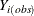and the variables with missing values by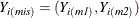, where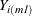is a subset of the missing variables that will cause a monotone missingness when their values are imputed. Then the I-step draws values forfrom a conditional distribution forgiven.

#### Posterior Step

The P-step is different from the P-step described in the section MCMC Method for Arbitrary Missing Multivariate Normal Data. Instead of simulating theand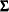parameters from the full imputed data set, this P-step simulates theandparameters through simulated regression coefficients from regression models based on the imputed data set with a monotone pattern of missingness. The step is similar to the process described in the section Monotone and FCS Regression Methods.

That is, for the variable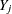, a model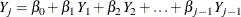is fitted usingnonmissing observations for variablein the imputed data sets.

The fitted model consists of the regression parameter estimates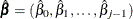and the associated covariance matrix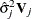, where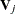is the usualinverse matrix from the intercept and variables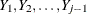.

For each imputation, new parameters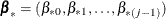and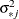are drawn from the posterior predictive distribution of the parameters. That is, they are simulated from,, and. The variance is drawn aswhere g is a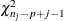random variate andis the number of nonmissing observations for. The regression coefficients are drawn as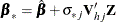whereis the upper triangular matrix in the Cholesky decomposition,, andis a vector of j independent random normal variates.

These simulated values ofandare then used to re-create the parametersand. For a detailed description of how to produce monotone missingness with the MCMC method for a multivariate normal data, see Schafer (1997, pp. 226–235).